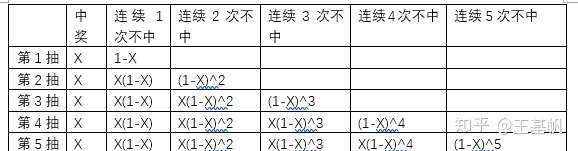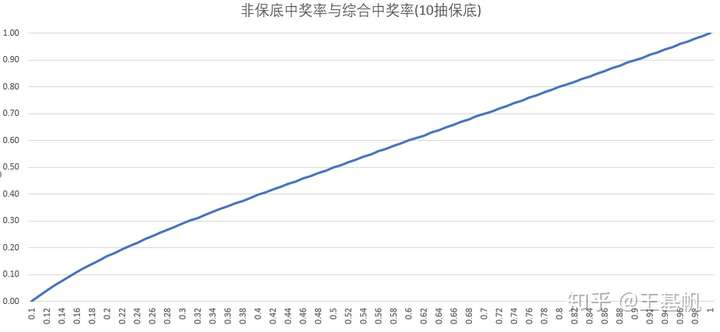# 游戏抽奖概率计算。不存在保底。但是可以计算

1383 2020-11-29 10:46

# 保底抽奖的概率计算方法，别再怪自己脸黑总是吃保底了！！

...

...public class Test {
public static void main(String[] args) {
double rate = findOriginalRate(0.15);
System.out.println(rate);
}

private static double findOriginalRate(double finalRate) {
double min = 0, max = 1, value = 0.5, rate = calculate(value);
do {
if (rate == finalRate) {
break;
}
if (rate < finalRate) {
min = (min + value) / 2;
} else {
max = (max + value) / 2;
}

value = (min + max) / 2;
// rate = simulate(value, 10000000);
rate = calculate(value);
} while (Math.abs(rate - finalRate) > 1E-6);
return value;
}

private static double calculate(double rate) {
double[][] record = new double;
record = 1;

int previousPos = 0;
for (int pos = 1;; pos = (pos + 1) % 10) {
record[pos] = 1;
for (int notHitCount = 1; notHitCount < 10; notHitCount++) {
record[pos][notHitCount] = record[previousPos][notHitCount - 1] * (1 - rate);
record[pos] -= record[pos][notHitCount];
}

previousPos = pos;

boolean shouldBreak = true;
for (int i = 1; i < 10; i++) {
if (Math.abs(record[i] - record) > 1E-10) {
shouldBreak = false;
break;
}
}
if (shouldBreak)
break;
}

return record;
}

private static double simulate(double rate, int times) {
int count = 0;
int normalHit = 0;
int finalHit = 0;
for (int i = 0; i < times; i++) {
if (count == 9) {
count = 0;
finalHit++;
continue;
}

if (Math.random() <= rate) {
count = 0;
normalHit++;
} else {
count++;
}
}

return (double) (normalHit + finalHit) / times;
}
}

simulate用于验证，毕竟模拟的程序是最好写的，calculate用于计算。·